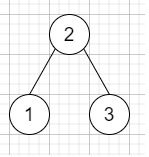# Inorder Successor in BST in C++

C++Server Side ProgrammingProgramming

Suppose we have a binary search tree and a node in it, we have to search the in-order successor of that node in the BST. As we know that the successor of a node p is the node with the smallest key greater than p.val.

So, if the input is like root = [2,1,3], p = 1,then the output will be 2,

To solve this, we will follow these steps −

• Define recursive method inorderSuccessor(), this will take root and p

• if root null, then −

• return null

• if val of root <= val of p, then −

• return inorderSuccessor(right of root , p)

• Otherwise

• option := inorderSuccessor(left of root , p)

• return (if option is zero, then root, otherwise option)

## Example

Let us see the following implementation to get a better understanding −

Live Demo

#include <bits/stdc++.h>
using namespace std;
class TreeNode{
public:
int val;
TreeNode *left, *right;
TreeNode(int data){
val = data;
left = NULL;
right = NULL;
}
};
class Solution {
public:
TreeNode* inorderSuccessor(TreeNode* root, TreeNode* p) {
if(!root) return NULL;
if(root->val <= p->val){
return inorderSuccessor(root->right, p);
}
else{
TreeNode* option = inorderSuccessor(root->left, p);
return !option ? root : option;
}
}
};
main(){
TreeNode *root = new TreeNode(2);
root->left = new TreeNode(1);
root->right = new TreeNode(3);
TreeNode *p = root->left;
Solution ob;
cout << (ob.inorderSuccessor(root, p))->val;
}

## Input

{2,1,3},1

## Output

2